# 其他

## 运行Python程序，提示找不到Python命令

``````c:\>python first.py
'python' 不是内部或外部命令，也不是可运行的程序

``````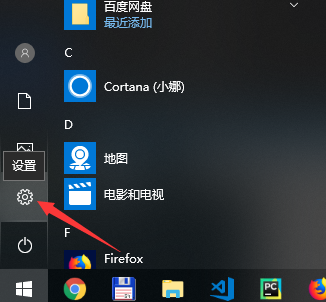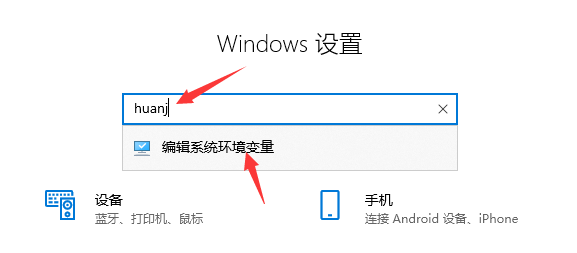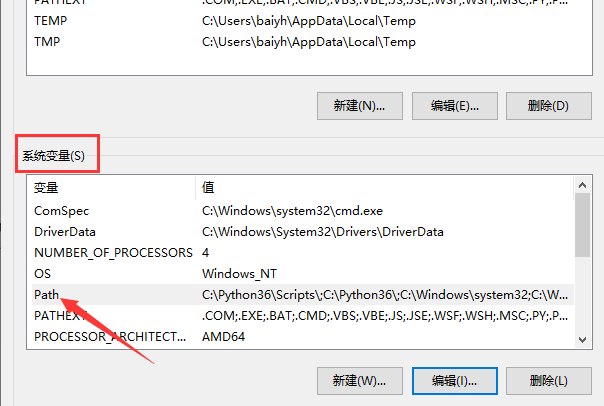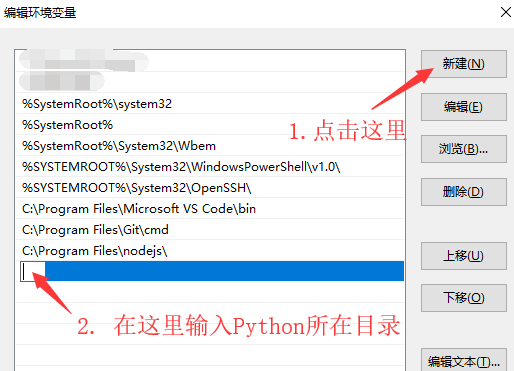• 你要看看你的python解释器安装在什么目录，填写你的目录。

• 一定是python所在目录 ，不要在最后加上python.exe

• 把python目录中的scripts目录也加上，就是最后要多两条记录，如下所示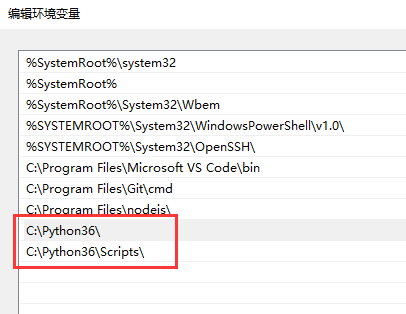## 运行Python程序，提示找不到代码文件

``````c:\>python first.py
python: can't open file 'first.py': [Errno 2] No such file or directory
``````

## 查看和修改当前工作目录

``````import os
curDir = os.getcwd()
``````

``````import os
os.chcwd('d:\\home')
``````

## 查看导入的模块的路径

``````>>> import selenium
>>> selenium.__file__
'C:\\Python36\\lib\\site-packages\\selenium\\__init__.py'
>>>
``````

## 查看当前正在执行的代码文件的路径

``````import os

# 当前代码文件路径
current_script_path = os.path.realpath( __file__ )
print(current_script_path)

# 代码文件所在目录路径
print(os.path.dirname(current_script_path))
``````

## 查看当前运行的python解释器的路径

``````import os,sys

pid_or_handle1 = os.spawnl(
os.P_NOWAIT,
sys.executable,
sys.executable,
"otherscript.py",
'param1',
'param2')
``````

## 判断一个对象是否可以执行

hasattr函数可以用来判断变量对应的对象是否是可执行的。

``````def obj1():
pass

obj2 = 'ok'

hasattr(obj1,'__call__') # 返回 True
hasattr(obj2,'__call__') # 返回 False

``````

## 字符串IP地址转换为 整数IP地址

``````import socket,struct

strIP = "192.168.0.1"
intIP = socket.ntohl(struct.unpack("I",socket.inet_aton(strIP)))
print(intIP)
``````

``````import socket,struct
intIP = 3232235521
strIP = socket.inet_ntoa(struct.pack('I',socket.htonl(intIP)))
print(strIP)
``````